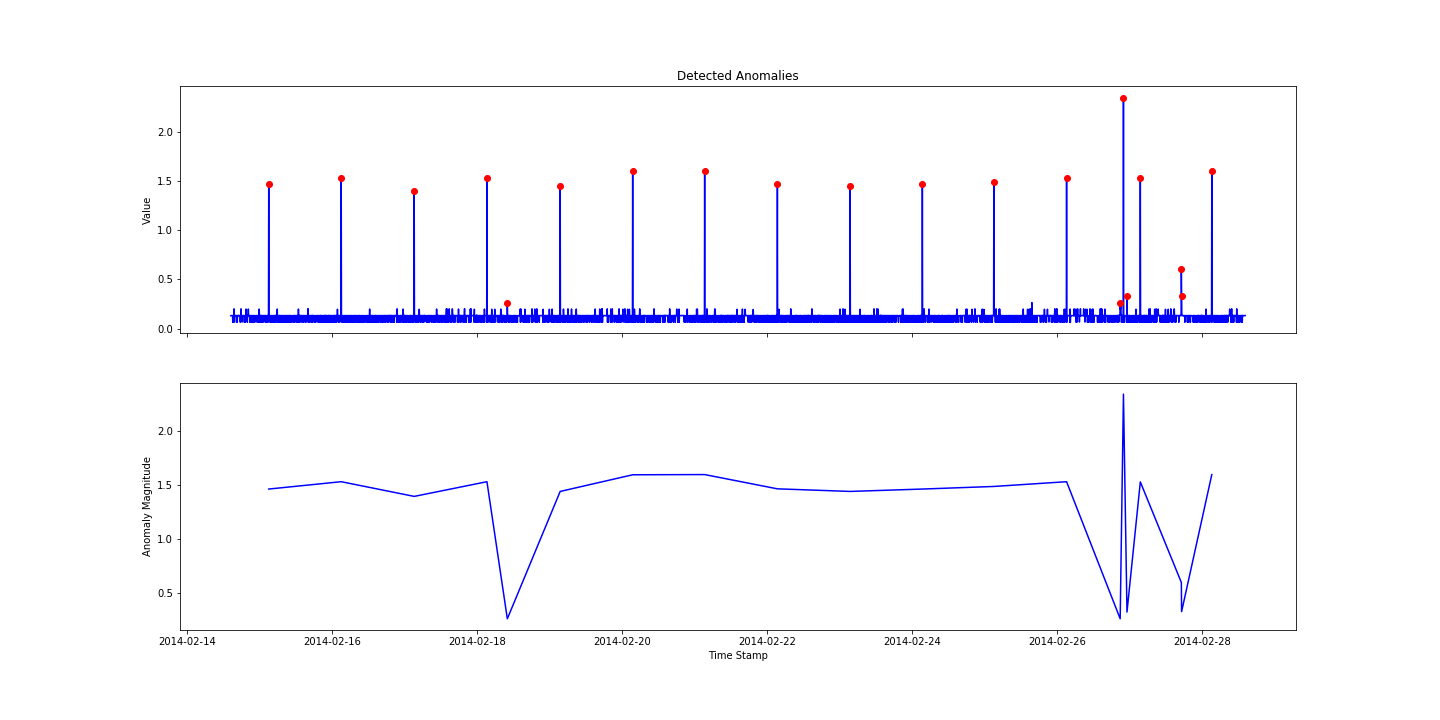# Outlier Detection with Twitter's Anomaly Detection

02 Jun 2018

Twitter’s Anomaly Detection was originally built in R, and python packages that reproduce it tend to require dependencies on R as well.

Well, not this one! Check out https://github.com/wdm0006/pyculiarity

Amazing stuff.

I downloaded some ec2 cpu utilisation data with anomalies from the Numenta Anomaly Benchmark (NAB) https://www.kaggle.com/boltzmannbrain/nab/data, pip installed pyculiar and BOOM - with a few lines of code:``````
import pandas as pd
import numpy as np
from pyculiarity import detect_ts
import matplotlib.pyplot as plt
%matplotlib inline

# convert timestamp string to datetime, then to unix timestamp integer
cpu_util['timestamp'] = pd.to_datetime(cpu_util['timestamp'])
cpu_util['timestamp'] = cpu_util['timestamp'].astype(np.int64) // 10**9

# run the model
results = detect_ts(cpu_util, max_anoms=0.005, alpha=0.001, direction='both')

# format the unix timestamp integer back to datetime
cpu_util['timestamp'] = pd.to_datetime(cpu_util['timestamp'])

# plot the graphs
f, ax = plt.subplots(2, 1, sharex=True, figsize=(20, 10))

ax.plot(cpu_util['timestamp'], cpu_util['value'], 'b')
ax.plot(results['anoms'].index, results['anoms']['anoms'], 'ro')
ax.set_title('Detected Anomalies')
ax.set_ylabel('Value')

ax.plot(results['anoms'].index, results['anoms']['anoms'], 'b')
ax.set_xlabel('Time Stamp')
ax.set_ylabel('Anomaly Magnitude')

# save the plot
plt.savefig('aws.png')

``````